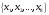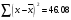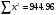# Chapter 3: intoduction to statistics the Statistical Process

 Page 6/6 Date 03.05.2017 Size 341.52 Kb. #18702

EXERCISE CHAPTER 3

SEM 3, 07/08

1. (a) Name the two main areas of statistics.

(b) State whether the variable from the following statements is discrete or continuous.

(i) The number of calls received by a switchboard operator each day for a month.

(ii) Lifetime (in hours) of 12 flashlight batteries.

(iii) Actual cost of a student’s textbook for a given semester.

1. (a) The following is the systolic blood pressure, in mm Hg, of 10 patients in a hospital

165 135 151 155 158 146 149 124 162 173

Find:

1. The number of patients whose systolic blood pressures exceed one standard score above or below the mean.

2. The data value that corresponds to the third quartile, Q3

(b) The table indicates the scores obtained by a group of students in a mathematics quiz.
 Score 0 1 2 3 4 5 Number of students 8 1 1 0 x 3

If the median is 1.

1. Find the value of x.

2. Hence, find the mode.

SEM 2, 07/08

1. State whether the following are nominal, ordinal, interval or ratio data.

1. A statistics test which a student took was easy, difficult or very difficult and these alternatives are coded 1, 2, and 3.

2. The temperature of different kilns at the factory.

3. The bottles on a Chemistry laboratory shelf are numbered 1,2,3 and 4 representing sulfuric acid, hydrochloric acid, nitric acid and sodium hydroxide.

4. The race of the students in a university campus.

1. (a) The mean of the ages of a group of 8 people is 40 years and the variance is 50 years2.

Two other people whose ages 30 years and 79 years join the group. Calculate the mean

and standard deviation of the ages of the 10 people.

(b) The number of hand phones that are sold in a week by 15 representatives in a town is

as follows:

5, 10, 8, 7, 25, 12, 5, 14, 11, 10, 21, 9,8 11,18

Find:

1. The number of representatives whose number of hand phones sold is above the median of the data set.

2. The data values that corresponds to the 63rd percentile.

1. The distribution of the weights of 133 mineral specimens collected on a field trip is given below:
 Weights (grams) Number of specimens 20-34 8 35-49 27 50-64 42 64-79 31 80-94 17 95-109 8

1. Find the median and mode.

2. Construct a percentile graph (use graph paper). Then, find:

1. The percentile rank for the weight of the mineral specimen of 45 grams.

2. The value of k if the weight of 25% of the specimens is at least k grams.

SEM 1, 07/08

1. State whether each of the following statements is true or false.

1. A study of statistics can be divided into two sections: qualitative and quantitative methods.

2. Ordinal scales permit comparison of scores or categories in terms of smaller or larger, higher or lower, or, best or worst.

3. The method of dividing the population elements into two groups based on income level and then selecting a simple random sample from each group is called cluster sampling.

4. The highest level of measurement is the interval level.

5. The weight of pumpkins is considered to be continuous variable.

1. (a) Given a set of numbers,, find

1. The variance of the set of numbers

2. The mean if1. The following data shows the number of television sets sold by a firm in period of 10 weeks :

15 21 5 6 7 29 9 10 14 12

Find the percentile rank for 10 television sets sold by a firm.

3.

1. The relative frequency distribution shown in the following table refers to the marks for statistics test obtained by a group of matriculation students. The mean for the distribution of marks is 51.9
 Marks 0-19 20-39 40-59 60-79 80-99 Relative Frequency 0.07 0.20 0.36 0.28 0.09

1. If the students consist of 55% male students and 45% female students and the mean obtained by male students are 51, find the mean marks obtained by the female students.

2. Draw an ogive using relative frequency.

3. If a student who scores 85 marks or more is given a grade A, estimate the percentage of students who obtained grade A.

4. Find D8.

SEM 3, 06/07

1. (a) Name the two areas of statistics.

(b) Identity each of the following as examples of qualitative or quantitative variables:

(i) The breaking strength of a given type of string.

(ii) The hair color of children auditioning for the theatre Ali Baba.

1. Whether or not a tap is defective.

2. The length of time required to answer a telephone call at an office.

1. (a) Farid scores 60 on an English test that has mean of 54 and a standard deviation of 3 and he scores 81 on a History test with a mean of 76 and a standard deviation of 2. On which test did he perform better? Give your reason.

(b) The following data shows the number of books sold by ABC publisher in the Kuala Lumpur Book Fair in a period of 11 days. Find the percentile rank of selling 12 books.

23 15 13 6 12 5

10 22 18 11 25

1. The income (in thousands of RM) of 28 managers, grouped by class mid-points are as follows:
 Mid-point 40 45 50 55 60 Number of managers 5 7 10 4 2

1. Construct a frequency distribution

2. Find the median

3. Find the standard deviation

4. Draw an ogive using relative frequency. (Use the graph paper) .

SEM 2, 06/07

1. (a) Classify the two groups of quantitative variables.

(b) State whether the data from the following statements is nominal, ordinal , interval or ratio.

(i) The normal operating temperature of a car engine.

(ii) Classification of students using an academic program.

1. Speaker of seminar rated as excellent, good, average or poor.

2. Number of hours parents spend with their children per day.

1. (a) The following data shows the number of television sets sold by a firm in a period of 9 weeks. Given that mode=m.

15 21 5 6 7 29 m 10 14

Find the possible value(s) of m if D7=m.

1. A statistics was conducted for 100 male students and 120 female students taking a pre-university course. The mean and standard deviation of the marks obtained by the male and female students are follows.
 Students Mean Standard deviation Male 55.8 6.5 Female 57.1 5.3

Calculate the mean and standard deviation of the marks obtained by all students.

Sem. 3, 07/08

1. (a) Descriptive , Inferential

(b) (i) Discrete (ii) Continuous (iii) Continuous

2. (a) (i) No. of patients = 7 (ii) Q3 = 162

(b) (i) x = 4 or 5 (ii) Mode = 0

Sem. 2, 07/08

1. (a) Nominal (b) Interval (c) Nominal (d) Nominal

2. (a) New mean = 42.9 ; Standard deviation = 14.48

(b) (i) Median =10 ; no. of representative = 7

(ii) P63 = 11

3. (a) median = 60.75 ; Mode = 58.15

(b) (i) 45 grams correspond to 20-21 percentile

(ii) Q3 ≈ 75-76 gm

Sem. 1, 07/08

1. (a) False (b) True (c) False (d) False (e) True

2. (a) (i) Variance = 6.58 (ii) Mean = 10.6

(b) P45 = 10

3. (b) (i) Mean female = 53 (iii) 6-7 ( iv) 70-72

Sem. 3 , 06/07

1. (a) (i) Descriptive , Inferential

(b) (i) Quantitative (ii) Qualitative ( iii) Qualitative (iv) Quantitative

2. (a) Farid did better in History since z-score is higher than English.

(b) P41 = 12

3. (b) Median = 48.5 (c) Standard Deviation = 5.78

Sem. 2, 06/07

1. (a) Continuous , Discrete

(b) (i) Interval (ii) Nominal (iii) Ordinal (iv) Ratio

2. (a) m = 15 or 21

(b) Mean = 56.51 ; Standard Deviation = 5.90